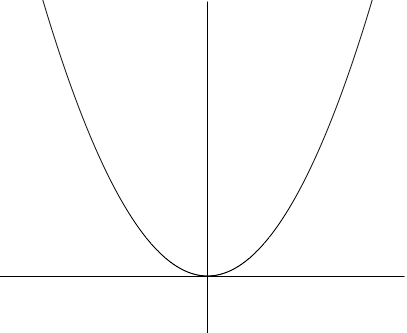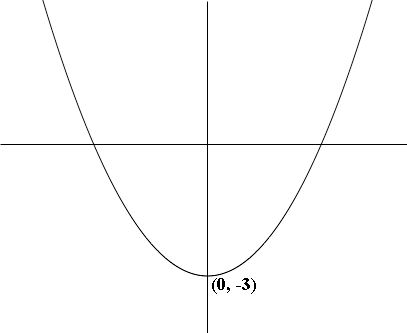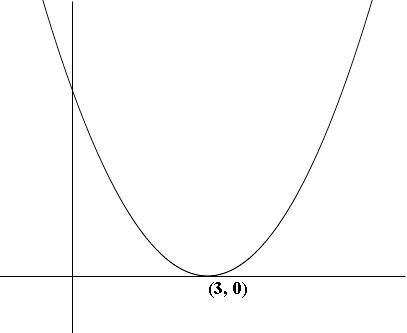SEARCH HOMEMath Central Quandaries & QueriesQuestion from Kapilan, a student: Please answer the following question: Sketch the graph of f(x)=1/x -2 Thank youKapilan,

I am going to illustrate with another function, g(x) = x2 - 3.

The technique is to plot h(x) = x2 and then look at how the graph changes when you subtract 3.

h(x) = x2 is I graph I expect you know.h(x) = x2

Subtracting 3 does not change the shape of the graph but it moves its position on the plane. For the function g(x) = x2 - 3 when x = 0, g(x) = -3 and hence the graph passes trough (0, -3). Hence its graph isg(x) = x2 - 3

What if you change the function h(x) = x2 by subtracting 3 from the input to the function, x, rather than the output h(x) to arrive at the function k(x) = (x - 3)2? Again you haven't changed the shape of the function, just where it sits in the plane. Here the vertex of the parabola is at x = 3 and hence the graph is shifted 3 units to the right and becomesk(x) = (x - 3)2

HarleyMath Central is supported by the University of Regina and The Pacific Institute for the Mathematical Sciences.